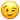# Puzzle: Sort Out The Men From Boys [reference]

“**Sort Out The Men From Boys **” is a coding puzzle that people can be attempted in the following languages: cpp, javascript, ruby, crystal, python, haskell, c, java, php, dart, julia, typescript, coffeescript, reason, elixir, racket, clojure, csharp, prolog, and cfml.

• Difficulty: 7 kyu
• Stars: 110
• Category: reference
• Tags: Fundamentals, Numbers, Data Types, Basic Language Features, Conditional Statements, Control Flow, Algorithms, Logic
• Source: codewars

# Scenario

Now that the competition gets tough it will Sort out the men from the boys .

Men are the Even numbers and Boys are the oddGiven an array/list [] of n integers , Separate The even numbers from the odds , or Separate the men from the boys# Notes

• Return an array/list where Even numbers come first then odds

• Since , Men are stronger than Boys , Then Even numbers in ascending order While odds in descending .

• Array/list size is at least 4 .

• Array/list numbers could be a mixture of positives , negatives .

• Have no fear , It is guaranteed that no Zeroes will exists .• Repetition of numbers in the array/list could occur , So (duplications are not included when separating).

# Input >> Output Examples:

``````
menFromBoys ({7, 3 , 14 , 17}) ==&gt; return ({14, 17, 7, 3})

``````

## Explanation:

Since , `{ 14 }` is the even number here , So it came first , then the odds in descending order `{17 , 7 , 3}` .

``````
menFromBoys ({-94, -99 , -100 , -99 , -96 , -99 }) ==&gt; return ({-100 , -96 , -94 , -99})

``````

## Explanation:

• Since , `{ -100, -96 , -94 }` is the even numbers here , So it came first in *ascending order *, then the odds in descending order `{ -99 }`

• Since , (Duplications are not included when separating) , then you can see only one (-99) was appeared in the final array/list .

``````
menFromBoys ({49 , 818 , -282 , 900 , 928 , 281 , -282 , -1 }) ==&gt; return ({-282 , 818 , 900 , 928 , 281 , 49 , -1})

``````

## Explanation:

• Since , `{-282 , 818 , 900 , 928 }` is the even numbers here , So it came first in ascending order , then the odds in descending order `{ 281 , 49 , -1 }`

• Since , (Duplications are not included when separating) , then you can see only one (-282) was appeared in the final array/list .

# Solve It Here

Click the link below to solve it on Codewars:

# Notes

This puzzle was posted by a Slackbot via a slash command. If you want to help work on the app, send a message to @Josh.

If you don’t want to see the coding puzzles when you visit the forum, you can go into your settings and mute the puzzles category.